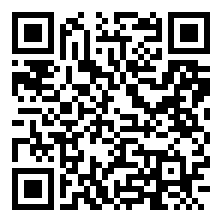『蓝桥杯』BASIC-3_字母图形IDforHYIT 2月 12, 2019• 在其它设备中阅读本文章
•『蓝桥杯』BASIC-3_字母图形

ABCDEFG

BABCDEF

CBABCDE

DCBABCD

EDCBABC

5 7

ABCDEFG
BABCDEF
CBABCDE
DCBABCD
EDCBABC

1 <= n, m <= 26。

ASCII码

# 代码：

``````#include<iostream>
#include<cmath>
using namespace std;
int main(){
int n=0, m=0;
cin >> n >> m;
for(int i=0; i<n; ++i){
for(int j=0; j<m; ++j){
cout << char('A'+ abs(i-j));
}
cout << endl;
}
return 0;
}
``````

This blog is under a CC BY-NC-SA 3.0 Unported License